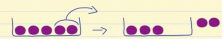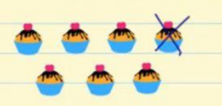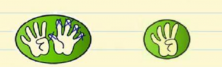Smartick is an advanced online program that teaches kids math and coding in only 15 min. a day

Aug25

# Learn How to Subtract with Examples

In this post, we are going to learn how to subtract.

First things first, we are going to learn exactly what subtraction is.

Subtraction is taking away a certain amount from another amount that we already have.

### Some examples of how to subtract:

If we have 5 purple balls in a box, and we take out 2 of these balls, there are 3 balls left in the box. This is:

## 5 – 2 = 3Here’s another example of subtraction: If we have 7 cakes and we eat 1 of them, how many cakes are we left with?

## 7 – 1 = 6Now, we’re going to learn how to subtract with our fingers. If we use 9 fingers—5 on one hand and 4 on the other— and we want to subtract 5 fingers, how many fingers are left? We need to hide the 5 fingers on one hand and count the ones that are left on the other. We’re left with 4 fingers. So:

## 9 – 5 = 4We hope you liked these elementary subtraction exercises! Share this post with your friends and family or leave a comment!

Check out other posts that teach subtraction: